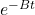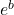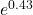Suppose a student recorded the following data during Step 10 of this experiment, A 6.2 deg 4.7 deg 3.2 deg 2.4 deg 1.8 deg

Question

Suppose a student recorded the following data during Step 10 of this experiment,

A 6.2 deg 4.7 deg 3.2 deg 2.4 deg 1.8 deg
Time 2 s 4 s 6 s 8 s 10 s
The student was careful to start taking data when the angular position of the pendulum was zero. Unfortunately he does not have a computer available to fit the data as you will in Step 12, but he knows that the amplitude of oscillations decays exponentially according to A= A0e-(Bt)+ C, where C = 1. Use the data obtained by the student to plot a straight line graph of the appropriate variables on the graph paper, and use it to find values for the parameters A0 and B.

in progress 0
5 months 2021-09-03T06:00:40+00:00 1 Answers 35 views 0

B = -0.215 s⁻¹,  A₀ = 1.537º

Explanation:

To be able to make this graph we must linearize the data, the best procedure is to calculate the logarithm of the values

A = A₀+ C

The constant creates a uniform displacement of the graph if we start the graph at the angle of 1, the constant disappears, this is done by subtracting 1 from each angle, so the equation is

(A-1) = A₀  e^{-Bt}

We do the logarithm

Log (A-1) = log Ao –Bt log e

Make this graph because paper commercially comes in logarithm 10, if we use graph paper if we can calculate directly in base logarithm e, let’s perform this calculation

Ln (A-1) = Ln A₀ – Bt

To graph the points we subtract 1 from each angle and calculate the logarithm, the data to be plotted are

θ’= ln (θ -1)

θ(º)    t(s)        θ'(º)

6.2      2     1.6487

4.7      4     1.3083

3.2      6    0.7885

2.4      8    0.3365

1.8     10   -0.2231

We can use a graph paper and graph on the axis and the primary angle (θ’) and on the x-axis time, mark the points and this graph is a straight line, we see that the point for greater time has a linearity deviation , so we will use the first three for the calculations

To find the line described by the equation

y -y₀ = m (x -x₀)

m = (y₂ -y₁) / (x₂ -x₁)

Where m is the slope of the graph e (x₀, y₀) is any point, let’s start as the first point of the series

(x₀, y₀) = (2, 1.65)

(x₂, y₂) = (2, 1.65)

(x₁, y₁) = (6, 0.789)

We use the slope equation

m = (1.65 – 0.789) / (2-6)

m = -0.215

The equation is

y – 1.65 = -0.215 (x- 2)

y = -0.215 x +0.4301

We buy the two equations and see that the slope is the constant B

B = -0.215 s⁻¹

The independent term is

b = ln A₀

A₀ =A₀ =A₀ = 1.537º